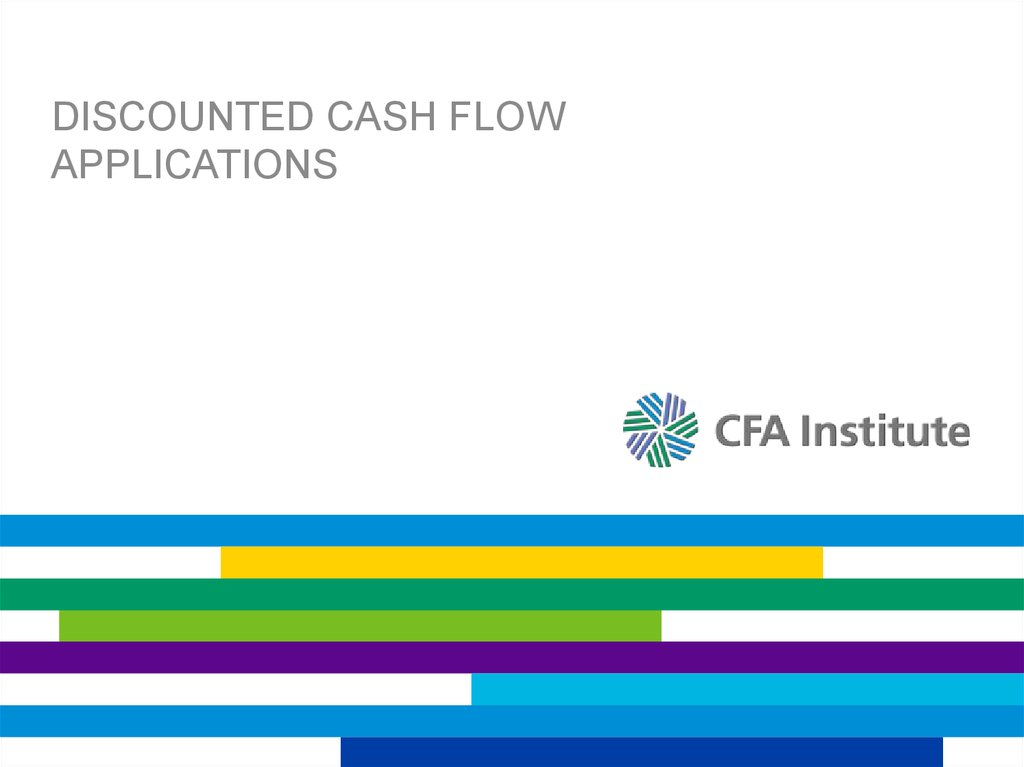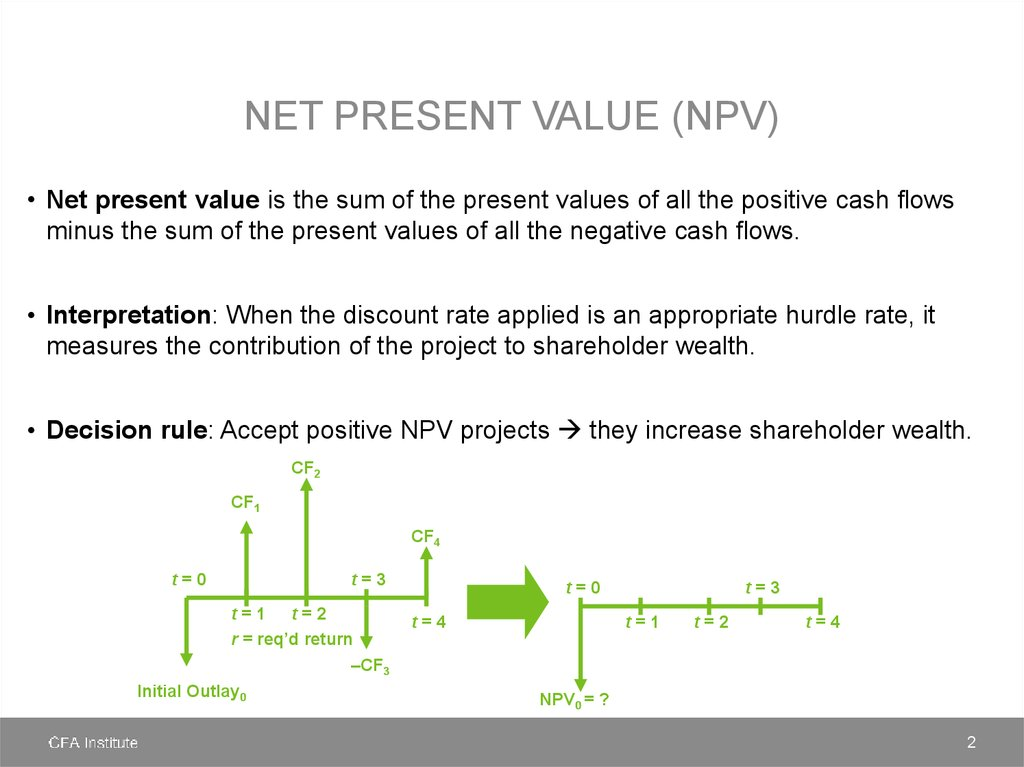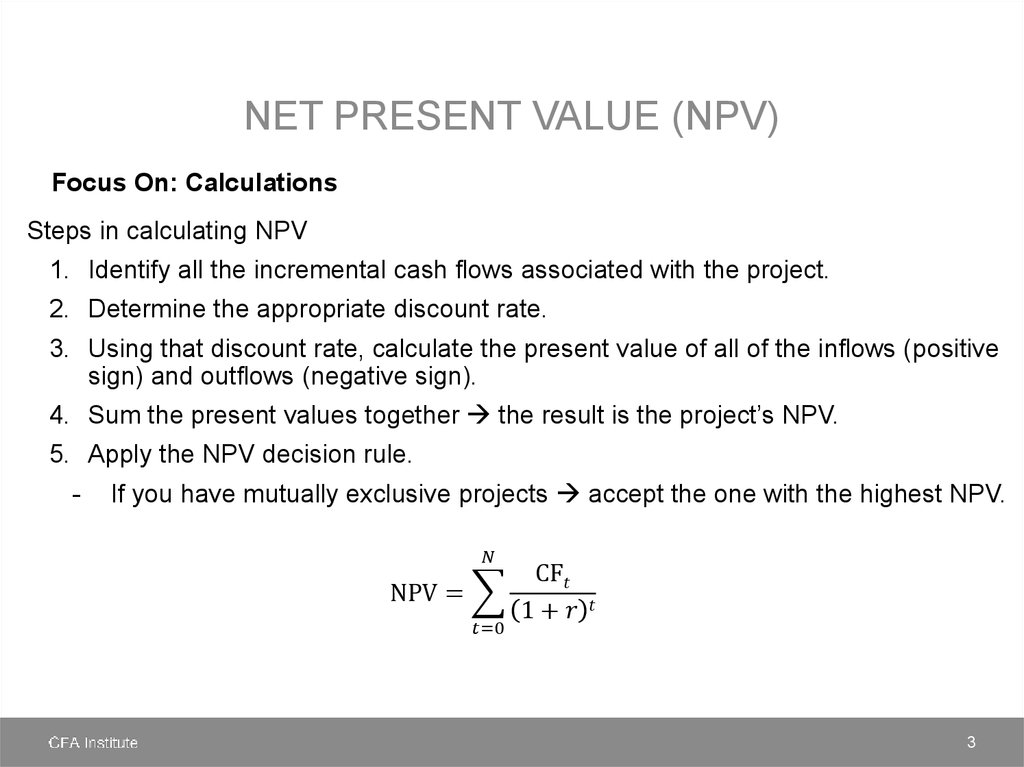# Discounted Cash Flow applications

## 1. Discounted Cash Flow applications

DISCOUNTED CASH FLOW
APPLICATIONS

## 2. Net present value (NPV)

NET PRESENT VALUE (NPV)
• Net present value is the sum of the present values of all the positive cash flows
minus the sum of the present values of all the negative cash flows.
• Interpretation: When the discount rate applied is an appropriate hurdle rate, it
measures the contribution of the project to shareholder wealth.
• Decision rule: Accept positive NPV projects they increase shareholder wealth.
CF2
CF1
CF4
t=0
t=3
t=1 t=2
r = req’d return
–CF3
Initial Outlay0
t=0
t=4
t=3
t=1
t=2
t=4
NPV0 = ?
2

## 3. Net present value (NPV)

NET PRESENT VALUE (NPV)
Focus On: Calculations
Steps in calculating NPV
1. Identify all the incremental cash flows associated with the project.
2. Determine the appropriate discount rate.
3. Using that discount rate, calculate the present value of all of the inflows (positive
sign) and outflows (negative sign).
4. Sum the present values together the result is the project’s NPV.
5. Apply the NPV decision rule.
-
If you have mutually exclusive projects accept the one with the highest NPV.